It is the kernel that I have tried and compiled from the courses of DATAI Team (Language of the courses is Turkish: Data Science ve Python: Sıfırdan Uzmanlığa Veri Bilimi (2) and Data Visualization: A'dan Z'ye Veri Görselleştirme (3)), which is Grandmaster on Kaggle and has more than 15 courses on Udemy.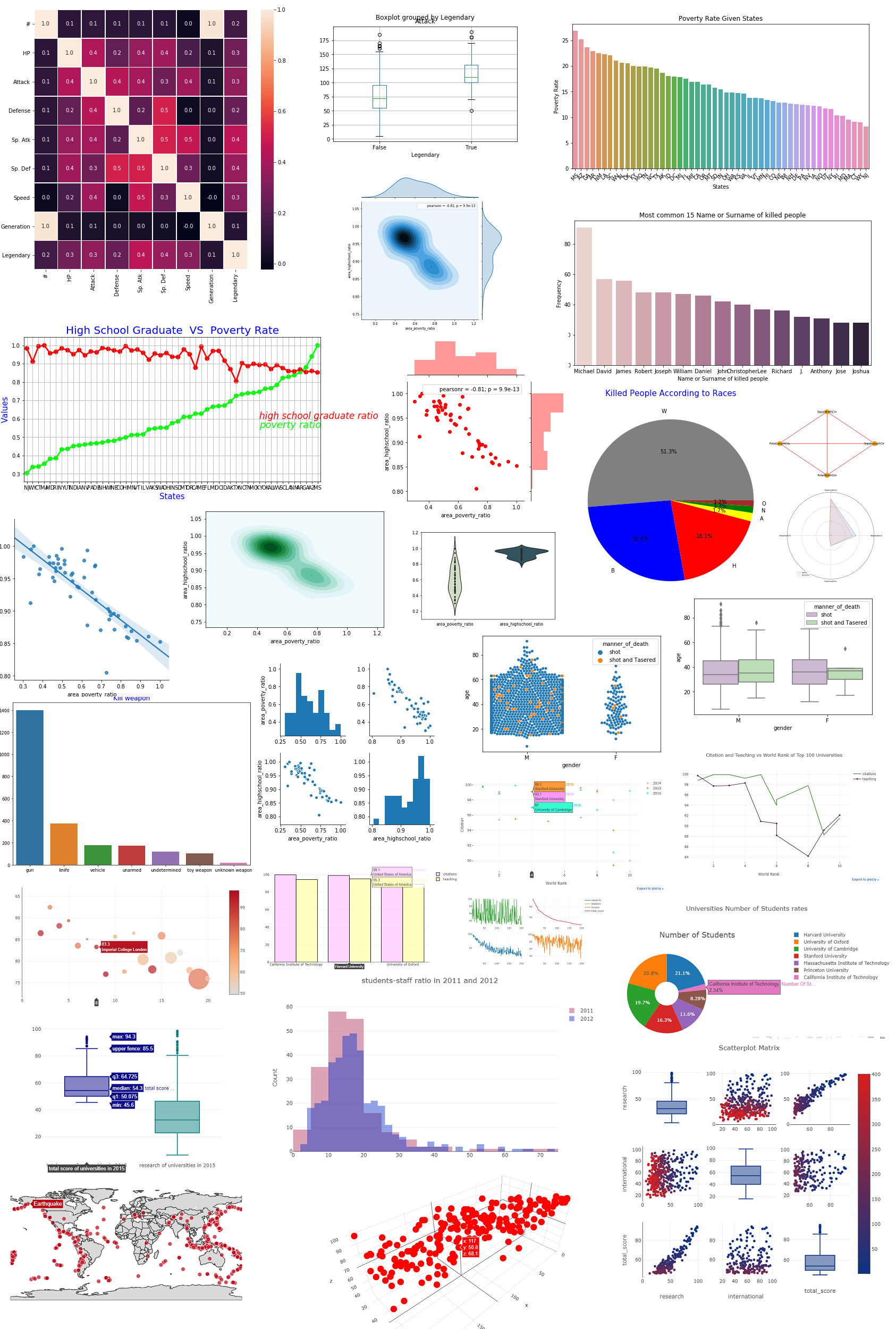# Diagnose data for cleaning¶

In :
import numpy as np # linear algebra
import pandas as pd # data processing, CSV file I/O (e.g. pd.read_csv)
import matplotlib.pyplot as plt
import seaborn as sns  # visualization tool
from subprocess import check_output
#print(check_output(["ls", "../input"]).decode("utf8"))
#data.info()

Out:
# Name Type 1 Type 2 HP Attack Defense Sp. Atk Sp. Def Speed Generation Legendary
0 1 Bulbasaur Grass Poison 45 49 49 65 65 45 1 False
1 2 Ivysaur Grass Poison 60 62 63 80 80 60 1 False
2 3 Venusaur Grass Poison 80 82 83 100 100 80 1 False
3 4 Mega Venusaur Grass Poison 80 100 123 122 120 80 1 False
4 5 Charmander Fire NaN 39 52 43 60 50 65 1 False
In :
data.tail()

Out:
# Name Type 1 Type 2 HP Attack Defense Sp. Atk Sp. Def Speed Generation Legendary
795 796 Diancie Rock Fairy 50 100 150 100 150 50 6 True
796 797 Mega Diancie Rock Fairy 50 160 110 160 110 110 6 True
797 798 Hoopa Confined Psychic Ghost 80 110 60 150 130 70 6 True
798 799 Hoopa Unbound Psychic Dark 80 160 60 170 130 80 6 True
799 800 Volcanion Fire Water 80 110 120 130 90 70 6 True
In :
data.columns

Out:
Index(['#', 'Name', 'Type 1', 'Type 2', 'HP', 'Attack', 'Defense', 'Sp. Atk',
'Sp. Def', 'Speed', 'Generation', 'Legendary'],
dtype='object')
In :
data.shape

Out:
(800, 12)
In :
data.info()

<class 'pandas.core.frame.DataFrame'>
RangeIndex: 800 entries, 0 to 799
Data columns (total 12 columns):
#             800 non-null int64
Name          799 non-null object
Type 1        800 non-null object
Type 2        414 non-null object
HP            800 non-null int64
Attack        800 non-null int64
Defense       800 non-null int64
Sp. Atk       800 non-null int64
Sp. Def       800 non-null int64
Speed         800 non-null int64
Generation    800 non-null int64
Legendary     800 non-null bool
dtypes: bool(1), int64(8), object(3)
memory usage: 69.6+ KB


# Exploratory data analysis (EDA)¶

In :
print(data['Type 1'].value_counts(dropna =False))  # if there are nan values that also be counted
# As it can be seen below there are 112 water pokemon or 70 grass pokemon

Water       112
Normal       98
Grass        70
Bug          69
Psychic      57
Fire         52
Electric     44
Rock         44
Dragon       32
Ground       32
Ghost        32
Dark         31
Poison       28
Fighting     27
Steel        27
Ice          24
Fairy        17
Flying        4
Name: Type 1, dtype: int64

In :
data.describe() #ignore null entries

Out:
# HP Attack Defense Sp. Atk Sp. Def Speed Generation
count 800.0000 800.000000 800.000000 800.000000 800.000000 800.000000 800.000000 800.00000
mean 400.5000 69.258750 79.001250 73.842500 72.820000 71.902500 68.277500 3.32375
std 231.0844 25.534669 32.457366 31.183501 32.722294 27.828916 29.060474 1.66129
min 1.0000 1.000000 5.000000 5.000000 10.000000 20.000000 5.000000 1.00000
25% 200.7500 50.000000 55.000000 50.000000 49.750000 50.000000 45.000000 2.00000
50% 400.5000 65.000000 75.000000 70.000000 65.000000 70.000000 65.000000 3.00000
75% 600.2500 80.000000 100.000000 90.000000 95.000000 90.000000 90.000000 5.00000
max 800.0000 255.000000 190.000000 230.000000 194.000000 230.000000 180.000000 6.00000
In :
data.corr()

Out:
# HP Attack Defense Sp. Atk Sp. Def Speed Generation Legendary
# 1.000000 0.097712 0.102664 0.094691 0.089199 0.085596 0.012181 0.983428 0.154336
HP 0.097712 1.000000 0.422386 0.239622 0.362380 0.378718 0.175952 0.058683 0.273620
Attack 0.102664 0.422386 1.000000 0.438687 0.396362 0.263990 0.381240 0.051451 0.345408
Defense 0.094691 0.239622 0.438687 1.000000 0.223549 0.510747 0.015227 0.042419 0.246377
Sp. Atk 0.089199 0.362380 0.396362 0.223549 1.000000 0.506121 0.473018 0.036437 0.448907
Sp. Def 0.085596 0.378718 0.263990 0.510747 0.506121 1.000000 0.259133 0.028486 0.363937
Speed 0.012181 0.175952 0.381240 0.015227 0.473018 0.259133 1.000000 -0.023121 0.326715
Generation 0.983428 0.058683 0.051451 0.042419 0.036437 0.028486 -0.023121 1.000000 0.079794
Legendary 0.154336 0.273620 0.345408 0.246377 0.448907 0.363937 0.326715 0.079794 1.000000
In :
#correlation map
f,ax = plt.subplots(figsize=(9, 9))
sns.heatmap(data.corr(), annot=True, linewidths=.5, fmt= '.1f',ax=ax)
plt.show()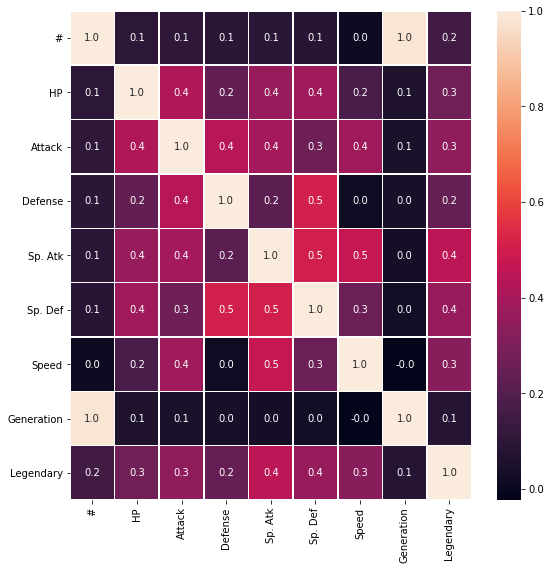# Visual exploratory data analysis¶

In :
data.boxplot(column='Attack',by = 'Legendary')
# For example: compare attack of pokemons that are legendary  or not
# Black line at top is max
# Blue line at top is 75%
# Red line is median (50%)
# Blue line at bottom is 25%
# Black line at bottom is min
# There are no outliers

Out:
<matplotlib.axes._subplots.AxesSubplot at 0x7f06c4ae0c18>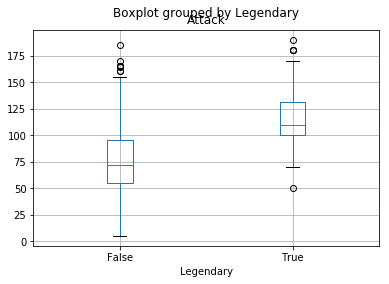# Tidy data¶

In :
data_new = data.head()    # I only take 5 rows into new data
data_new

Out:
# Name Type 1 Type 2 HP Attack Defense Sp. Atk Sp. Def Speed Generation Legendary
0 1 Bulbasaur Grass Poison 45 49 49 65 65 45 1 False
1 2 Ivysaur Grass Poison 60 62 63 80 80 60 1 False
2 3 Venusaur Grass Poison 80 82 83 100 100 80 1 False
3 4 Mega Venusaur Grass Poison 80 100 123 122 120 80 1 False
4 5 Charmander Fire NaN 39 52 43 60 50 65 1 False
In :
# lets melt
# id_vars = what we do not wish to melt
# value_vars = what we want to melt
melted = pd.melt(frame=data_new,id_vars = 'Name', value_vars= ['Attack','Defense'])
melted

Out:
Name variable value
0 Bulbasaur Attack 49
1 Ivysaur Attack 62
2 Venusaur Attack 82
3 Mega Venusaur Attack 100
4 Charmander Attack 52
5 Bulbasaur Defense 49
6 Ivysaur Defense 63
7 Venusaur Defense 83
8 Mega Venusaur Defense 123
9 Charmander Defense 43

# Pivoting data¶

In :
# Index is name
# I want to make that columns are variable
# Finally values in columns are value
melted.pivot(index = 'Name', columns = 'variable',values='value')

Out:
variable Attack Defense
Name
Bulbasaur 49 49
Charmander 52 43
Ivysaur 62 63
Mega Venusaur 100 123
Venusaur 82 83

# Concatenating data¶

In :
# Firstly lets create 2 data frame
data2= data.tail()
conc_data_row = pd.concat([data1,data2],axis =0,ignore_index =True) # axis = 0 : adds dataframes in row
conc_data_row

Out:
# Name Type 1 Type 2 HP Attack Defense Sp. Atk Sp. Def Speed Generation Legendary
0 1 Bulbasaur Grass Poison 45 49 49 65 65 45 1 False
1 2 Ivysaur Grass Poison 60 62 63 80 80 60 1 False
2 3 Venusaur Grass Poison 80 82 83 100 100 80 1 False
3 4 Mega Venusaur Grass Poison 80 100 123 122 120 80 1 False
4 5 Charmander Fire NaN 39 52 43 60 50 65 1 False
5 796 Diancie Rock Fairy 50 100 150 100 150 50 6 True
6 797 Mega Diancie Rock Fairy 50 160 110 160 110 110 6 True
7 798 Hoopa Confined Psychic Ghost 80 110 60 150 130 70 6 True
8 799 Hoopa Unbound Psychic Dark 80 160 60 170 130 80 6 True
9 800 Volcanion Fire Water 80 110 120 130 90 70 6 True
In :
data1 = data['Attack'].head()
conc_data_col = pd.concat([data1,data2],axis =1) # axis = 0 : adds dataframes in row
conc_data_col

Out:
Attack Defense
0 49 49
1 62 63
2 82 83
3 100 123
4 52 43

# Data types¶

In :
print(data.dtypes)
#data['Type 1'] = data['Type 1'].astype('category')
#data['Speed'] = data['Speed'].astype('float')
#print(data.dtypes)

#              int64
Name          object
Type 1        object
Type 2        object
HP             int64
Attack         int64
Defense        int64
Sp. Atk        int64
Sp. Def        int64
Speed          int64
Generation     int64
Legendary       bool
dtype: object


# Missing data and testing with assert¶

In :
# Lets chech Type 2
data["Type 2"].value_counts(dropna =False)
# As you can see, there are 386 NAN value

Out:
NaN         386
Flying       97
Ground       35
Poison       34
Psychic      33
Fighting     26
Grass        25
Fairy        23
Steel        22
Dark         20
Dragon       18
Ghost        14
Rock         14
Ice          14
Water        14
Fire         12
Electric      6
Normal        4
Bug           3
Name: Type 2, dtype: int64
In :
# Lets drop nan values
data1=data.copy()   # also we will use data to fill missing value so I assign it to data1 variable
data1["Type 2"].dropna(inplace = True)  # inplace = True means we do not assign it to new variable. Changes automatically assigned to data
assert  data1['Type 2'].notnull().all() # returns nothing because we drop nan values
data1["Type 2"].fillna('empty',inplace = True) # ıstersen empty ıle de doldurabılırız
# # With assert statement we can check a lot of thing. For example
# assert data.columns == 'Name'
# assert data.Speed.dtypes == np.int


# Index objects and labeled data¶

In :
# We can make one of the column as index. I actually did it at the beginning of manipulating data frames with pandas section
# It was like this
data= data.set_index("#")
# also you can use
# data.index = data["#"]
print(data.index.name)
data.index.name = "index_name" # lets change it

#

Out:
Name Type 1 Type 2 HP Attack Defense Sp. Atk Sp. Def Speed Generation Legendary
index_name
1 Bulbasaur Grass Poison 45 49 49 65 65 45 1 False
2 Ivysaur Grass Poison 60 62 63 80 80 60 1 False
3 Venusaur Grass Poison 80 82 83 100 100 80 1 False
4 Mega Venusaur Grass Poison 80 100 123 122 120 80 1 False
5 Charmander Fire NaN 39 52 43 60 50 65 1 False
In :
# Overwrite index
# if we want to modify index we need to change all of them.
# first copy of our data to data3 then change index
data3 = data.copy()
# lets make index start from 100. It is not remarkable change but it is just example
data3.index = range(100,900,1)

Out:
Name Type 1 Type 2 HP Attack Defense Sp. Atk Sp. Def Speed Generation Legendary
100 Bulbasaur Grass Poison 45 49 49 65 65 45 1 False
101 Ivysaur Grass Poison 60 62 63 80 80 60 1 False
102 Venusaur Grass Poison 80 82 83 100 100 80 1 False
103 Mega Venusaur Grass Poison 80 100 123 122 120 80 1 False
104 Charmander Fire NaN 39 52 43 60 50 65 1 False

# Hierarchical indexing¶

In :
# Setting index : type 1 is outer type 2 is inner index
data1 = data.set_index(["Type 1","Type 2"])
# data1.loc["Fire","Flying"] # howw to use indexes

Out:
Name HP Attack Defense Sp. Atk Sp. Def Speed Generation Legendary
Type 1 Type 2
Grass Poison Bulbasaur 45 49 49 65 65 45 1 False
Poison Ivysaur 60 62 63 80 80 60 1 False
Poison Venusaur 80 82 83 100 100 80 1 False
Poison Mega Venusaur 80 100 123 122 120 80 1 False
Fire NaN Charmander 39 52 43 60 50 65 1 False
NaN Charmeleon 58 64 58 80 65 80 1 False
Flying Charizard 78 84 78 109 85 100 1 False
Dragon Mega Charizard X 78 130 111 130 85 100 1 False
Flying Mega Charizard Y 78 104 78 159 115 100 1 False
Water NaN Squirtle 44 48 65 50 64 43 1 False

# Pivoting data frames¶

In :
dic = {"treatment":["A","A","B","B"],"gender":["F","M","F","M"],"response":[10,45,5,9],"age":[15,4,72,65]}
df = pd.DataFrame(dic)
df

Out:
treatment gender response age
0 A F 10 15
1 A M 45 4
2 B F 5 72
3 B M 9 65
In :
# pivoting
df.pivot(index="treatment",columns = "gender",values="response")

Out:
gender F M
treatment
A 10 45
B 5 9

# Stacking and unstacking data frames¶

In :
df1 = df.set_index(["treatment","gender"])
df1
# lets unstack it

Out:
response age
treatment gender
A F 10 15
M 45 4
B F 5 72
M 9 65
In :
# level determines indexes
df1.unstack(level=0)

Out:
response age
treatment A B A B
gender
F 10 5 15 72
M 45 9 4 65
In :
df1.unstack(level=1)

Out:
response age
gender F M F M
treatment
A 10 45 15 4
B 5 9 72 65
In :
# change inner and outer level index position
df2 = df1.swaplevel(0,1)
df2

Out:
response age
gender treatment
F A 10 15
M A 45 4
F B 5 72
M B 9 65

# Melting data frames¶

In :
df

Out:
treatment gender response age
0 A F 10 15
1 A M 45 4
2 B F 5 72
3 B M 9 65
In :
# df.pivot(index="treatment",columns = "gender",values="response")
pd.melt(df,id_vars="treatment",value_vars=["age","response"])

Out:
treatment variable value
0 A age 15
1 A age 4
2 B age 72
3 B age 65
4 A response 10
5 A response 45
6 B response 5
7 B response 9

# Categoricals and groupby¶

In :
# We will use df
df

Out:
treatment gender response age
0 A F 10 15
1 A M 45 4
2 B F 5 72
3 B M 9 65
In :
#according to treatment take means of other features
df.groupby("treatment").mean()   # mean is aggregation / reduction method
# there are other methods like sum, std,max or min

Out:
response age
treatment
A 27.5 9.5
B 7.0 68.5
In :
# we can only choose one of the feature
df.groupby("treatment").age.max()

Out:
treatment
A    15
B    72
Name: age, dtype: int64
In :
df.groupby("treatment")[["age","response"]].min()

Out:
age response
treatment
A 4 10
B 65 5

# Bar Plot¶

In :
import numpy as np # linear algebra
import pandas as pd # data processing, CSV file I/O (e.g. pd.read_csv)
import seaborn as sns
import matplotlib.pyplot as plt
from collections import Counter
%matplotlib inline


In :
percentage_people_below_poverty_level.head()

Out:
Geographic Area City poverty_rate
0 AL Abanda CDP 78.8
1 AL Abbeville city 29.1
4 AL Akron town 42
In :
# Poverty rate of each state
percentage_people_below_poverty_level.poverty_rate.replace(['-'],0.0,inplace = True)
percentage_people_below_poverty_level.poverty_rate = percentage_people_below_poverty_level.poverty_rate.astype(float)
area_list = list(percentage_people_below_poverty_level['Geographic Area'].unique())
area_poverty_ratio = []
for i in area_list:
x = percentage_people_below_poverty_level[percentage_people_below_poverty_level['Geographic Area']==i]
area_poverty_rate = sum(x.poverty_rate)/len(x)
area_poverty_ratio.append(area_poverty_rate)
data = pd.DataFrame({'area_list': area_list,'area_poverty_ratio':area_poverty_ratio})
new_index = (data['area_poverty_ratio'].sort_values(ascending=False)).index.values
sorted_data = data.reindex(new_index)

# visualization
plt.figure(figsize=(10,5))
sns.barplot(x=sorted_data['area_list'], y=sorted_data['area_poverty_ratio'])
plt.xticks(rotation= 45)
plt.xlabel('States')
plt.ylabel('Poverty Rate')
plt.title('Poverty Rate Given States')

Out:
Text(0.5,1,'Poverty Rate Given States')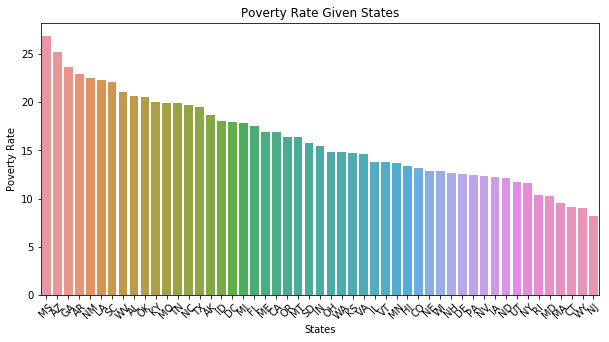In :
kill.head()

Out:
id name date manner_of_death armed age gender race city state signs_of_mental_illness threat_level flee body_camera
0 3 Tim Elliot 02/01/15 shot gun 53.0 M A Shelton WA True attack Not fleeing False
1 4 Lewis Lee Lembke 02/01/15 shot gun 47.0 M W Aloha OR False attack Not fleeing False
2 5 John Paul Quintero 03/01/15 shot and Tasered unarmed 23.0 M H Wichita KS False other Not fleeing False
3 8 Matthew Hoffman 04/01/15 shot toy weapon 32.0 M W San Francisco CA True attack Not fleeing False
4 9 Michael Rodriguez 04/01/15 shot nail gun 39.0 M H Evans CO False attack Not fleeing False
In :
# Most common 15 Name or Surname of killed people
separate = kill.name[kill.name != 'TK TK'].str.split()
a,b = zip(*separate)
name_list = a+b
name_count = Counter(name_list)
most_common_names = name_count.most_common(15)
x,y = zip(*most_common_names)
x,y = list(x),list(y)
#
plt.figure(figsize=(10,5))
ax= sns.barplot(x=x, y=y,palette = sns.cubehelix_palette(len(x)))
plt.xlabel('Name or Surname of killed people')
plt.ylabel('Frequency')
plt.title('Most common 15 Name or Surname of killed people')

Out:
Text(0.5,1,'Most common 15 Name or Surname of killed people')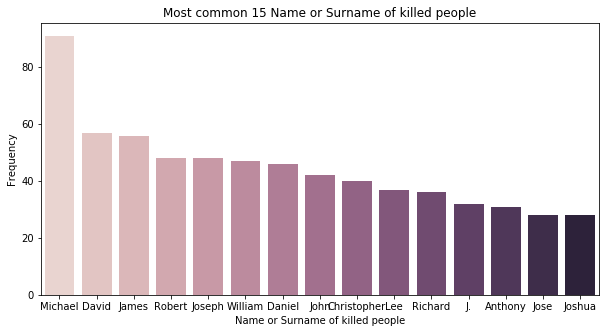# Point Plot¶

In :
percent_over_25_completed_highSchool.percent_completed_hs.replace(['-'],0.0,inplace = True)
percent_over_25_completed_highSchool.percent_completed_hs = percent_over_25_completed_highSchool.percent_completed_hs.astype(float)
area_list = list(percent_over_25_completed_highSchool['Geographic Area'].unique())
area_highschool = []
for i in area_list:
x = percent_over_25_completed_highSchool[percent_over_25_completed_highSchool['Geographic Area']==i]
area_highschool_rate = sum(x.percent_completed_hs)/len(x)
area_highschool.append(area_highschool_rate)
# sorting
data = pd.DataFrame({'area_list': area_list,'area_highschool_ratio':area_highschool})
new_index = (data['area_highschool_ratio'].sort_values(ascending=True)).index.values
sorted_data2 = data.reindex(new_index)

In :
# high school graduation rate vs Poverty rate of each state
sorted_data['area_poverty_ratio'] = sorted_data['area_poverty_ratio']/max( sorted_data['area_poverty_ratio'])
sorted_data2['area_highschool_ratio'] = sorted_data2['area_highschool_ratio']/max( sorted_data2['area_highschool_ratio'])
data = pd.concat([sorted_data,sorted_data2['area_highschool_ratio']],axis=1)
data.sort_values('area_poverty_ratio',inplace=True)

# visualize
f,ax1 = plt.subplots(figsize =(10,5))
sns.pointplot(x='area_list',y='area_poverty_ratio',data=data,color='lime',alpha=0.8)
sns.pointplot(x='area_list',y='area_highschool_ratio',data=data,color='red',alpha=0.8)
plt.text(40,0.6,'high school graduate ratio',color='red',fontsize = 17,style = 'italic')
plt.text(40,0.55,'poverty ratio',color='lime',fontsize = 18,style = 'italic')
plt.xlabel('States',fontsize = 15,color='blue')
plt.ylabel('Values',fontsize = 15,color='blue')
plt.title('High School Graduate  VS  Poverty Rate',fontsize = 20,color='blue')
plt.grid()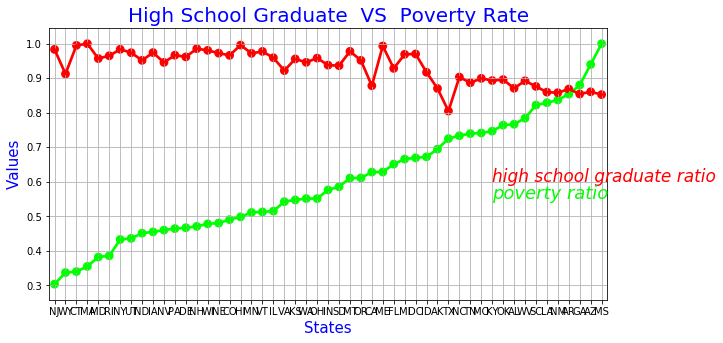# Joint Plot¶

In :
# Visualization of high school graduation rate vs Poverty rate of each state with different style of seaborn code
# joint kernel density
# pearsonr= if it is 1, there is positive correlation and if it is, -1 there is negative correlation.
# If it is zero, there is no correlation between variables
# Show the joint distribution using kernel density estimation
g = sns.jointplot(data.area_poverty_ratio, data.area_highschool_ratio, kind="kde", size=7)
plt.savefig('graph.png')
plt.show()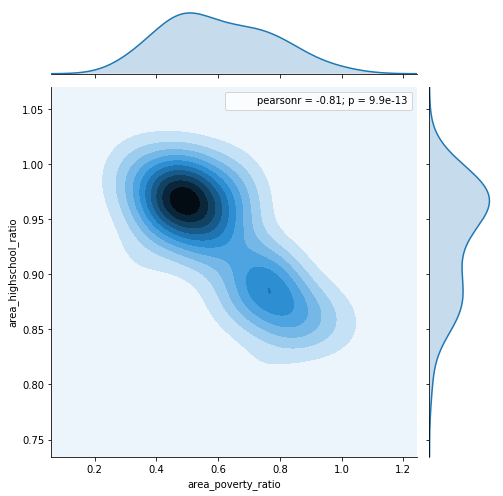In :
# you can change parameters of joint plot
# kind : { “scatter” | “reg” | “resid” | “kde” | “hex” }
# Different usage of parameters but same plot with previous one
g = sns.jointplot("area_poverty_ratio", "area_highschool_ratio", data=data,size=5, ratio=3, color="r")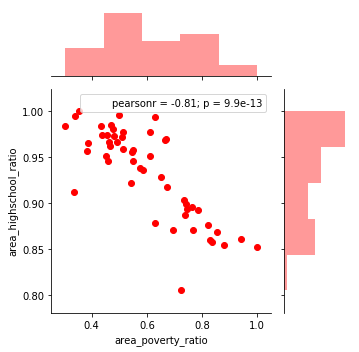# Pie Plot¶

In :
# Race rates according in kill data
kill.race.dropna(inplace = True)
labels = kill.race.value_counts().index
colors = ['grey','blue','red','yellow','green','brown']
explode = [0,0,0,0,0,0]
sizes = kill.race.value_counts().values

# visual
plt.figure(figsize = (7,7))
plt.pie(sizes, explode=explode, labels=labels, colors=colors, autopct='%1.1f%%')
plt.title('Killed People According to Races',color = 'blue',fontsize = 15)

Out:
Text(0.5,1,'Killed People According to Races')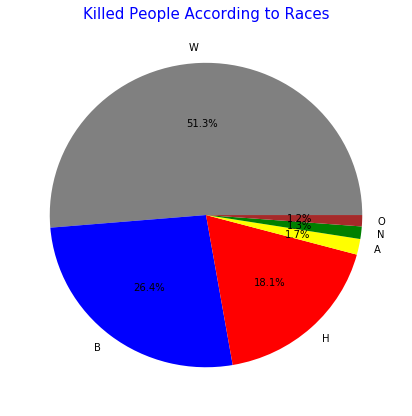# Lm Plot¶

In :
data.head()

Out:
area_list area_poverty_ratio area_highschool_ratio
30 NJ 0.303558 0.983615
50 WY 0.337139 0.912479
6 CT 0.339883 0.995254
21 MA 0.355090 1.000000
20 MD 0.382097 0.957107
In :
# Visualization of high school graduation rate vs Poverty rate of each state with different style of seaborn code
# lmplot
# Show the results of a linear regression within each dataset
sns.lmplot(x="area_poverty_ratio", y="area_highschool_ratio", data=data)
plt.show()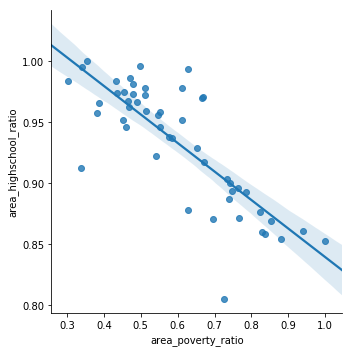# Kde Plot¶

In :
sns.kdeplot(data.area_poverty_ratio, data.area_highschool_ratio, shade=True, cut=3)
plt.show()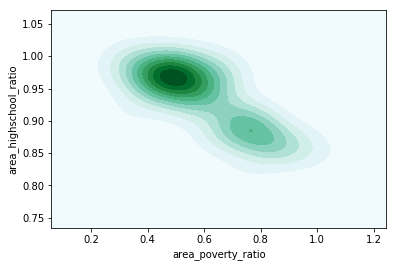# Violin Plot¶

In :
# Show each distribution with both violins and points
# Use cubehelix to get a custom sequential palette
pal = sns.cubehelix_palette(2, rot=-.5, dark=.3)
sns.violinplot(data=data, palette=pal, inner="points")
plt.show()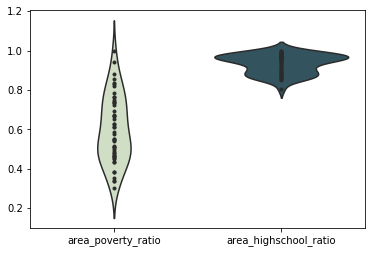# Heatmap¶

In :


In :
#correlation map
# Visualization of high school graduation rate vs Poverty rate of each state with different style of seaborn code
f,ax = plt.subplots(figsize=(5, 5))
sns.heatmap(data.corr(), annot=True, linewidths=0.5,linecolor="red", fmt= '.1f',ax=ax)
plt.show()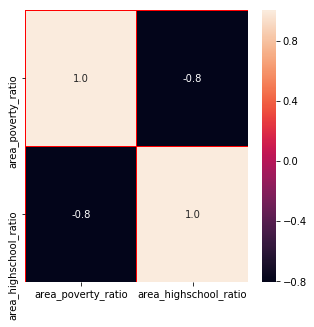# Box Plot¶

In :
# manner of death(olum sekli) : ates edilerek, ates edilerek ve sok tabancasiyla
# gender cinsiyet
# age: yas
# Plot the orbital period with horizontal boxes
sns.boxplot(x="gender", y="age", hue="manner_of_death", data=kill, palette="PRGn")
plt.show()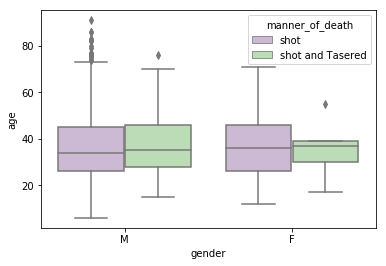# Swarm Plot¶

In :
# swarm plot
# manner of death(olum sekli) : ates edilerek, ates edilerek ve sok tabancasiyla
# gender cinsiyet
# age: yas
sns.swarmplot(x="gender", y="age",hue="manner_of_death", data=kill)
plt.show()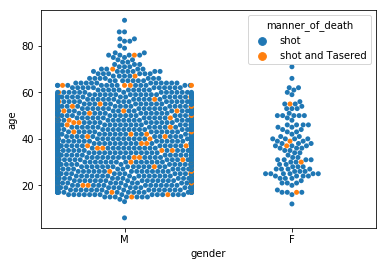# Pair Plot¶

In :
# pair plot
sns.pairplot(data)
plt.show()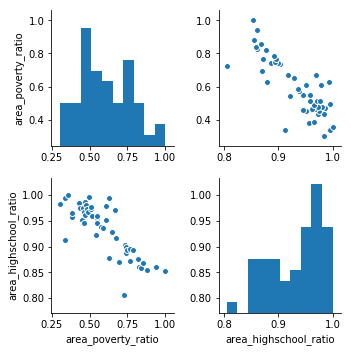# Count Plot¶

In :
# kill properties
# Manner of death
sns.countplot(kill.gender)
#sns.countplot(kill.manner_of_death)
plt.title("gender",color = 'blue',fontsize=15)

Out:
Text(0.5,1,'gender')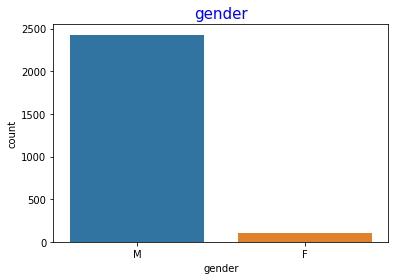In :
# kill weapon
armed = kill.armed.value_counts()
#print(armed)
plt.figure(figsize=(10,7))
sns.barplot(x=armed[:7].index,y=armed[:7].values)
plt.ylabel('Number of Weapon')
plt.xlabel('Weapon Types')
plt.title('Kill weapon',color = 'blue',fontsize=15)

Out:
Text(0.5,1,'Kill weapon')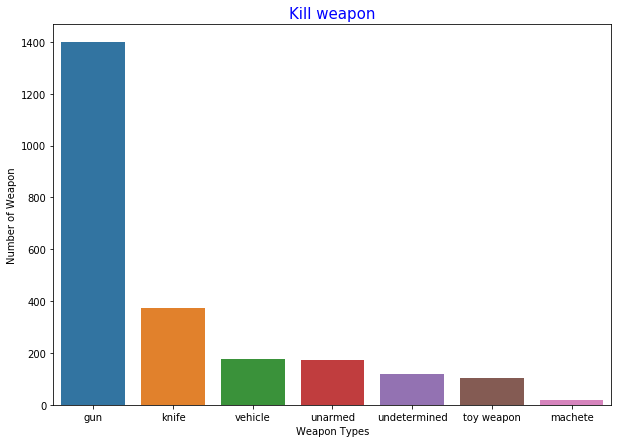# Line Plot¶

In :
# This Python 3 environment comes with many helpful analytics libraries installed
# It is defined by the kaggle/python docker image: https://github.com/kaggle/docker-python

import numpy as np # linear algebra
import pandas as pd # data processing, CSV file I/O (e.g. pd.read_csv)

# plotly
import plotly.plotly as py
from plotly.offline import init_notebook_mode, iplot
init_notebook_mode(connected=True)
import plotly.graph_objs as go

# word cloud library
from wordcloud import WordCloud

# matplotlib
import matplotlib.pyplot as plt
# Input data files are available in the "../input/" directory.
# For example, running this (by clicking run or pressing Shift+Enter) will list the files in the input directory

# Load data that we will use.

Out:
world_rank university_name country teaching international research citations income total_score num_students student_staff_ratio international_students female_male_ratio year
0 1 Harvard University United States of America 99.7 72.4 98.7 98.8 34.5 96.1 20,152 8.9 25% NaN 2011
1 2 California Institute of Technology United States of America 97.7 54.6 98.0 99.9 83.7 96.0 2,243 6.9 27% 33 : 67 2011
2 3 Massachusetts Institute of Technology United States of America 97.8 82.3 91.4 99.9 87.5 95.6 11,074 9.0 33% 37 : 63 2011
3 4 Stanford University United States of America 98.3 29.5 98.1 99.2 64.3 94.3 15,596 7.8 22% 42 : 58 2011
4 5 Princeton University United States of America 90.9 70.3 95.4 99.9 - 94.2 7,929 8.4 27% 45 : 55 2011
In :
# prepare data frame
df = timesData.iloc[:10,:]

# import graph objects as "go"
import plotly.graph_objs as go

# Creating trace1
trace1 = go.Scatter(
x = df.world_rank,
y = df.citations,
mode = "lines",
name = "citations",
marker = dict(color = 'rgba(16, 112, 2, 0.8)'),
text= df.university_name)
# Creating trace2
trace2 = go.Scatter(
x = df.world_rank,
y = df.teaching,
mode = "lines+markers",
name = "teaching",
marker = dict(color = 'rgba(80, 26, 80, 0.8)'),
text= df.university_name)
data = [trace1, trace2]
layout = dict(title = 'Citation and Teaching vs World Rank of Top 100 Universities',
xaxis= dict(title= 'World Rank',ticklen= 5,zeroline= False)
)
fig = dict(data = data, layout = layout)
iplot(fig)


# Scatter Plot¶

In :
# prepare data frames
df2014 = timesData[timesData.year == 2014].iloc[:10,:]
df2015 = timesData[timesData.year == 2015].iloc[:10,:]
df2016 = timesData[timesData.year == 2016].iloc[:10,:]
# import graph objects as "go"
import plotly.graph_objs as go
# creating trace1
trace1 =go.Scatter(
x = df2014.world_rank,
y = df2014.citations,
mode = "markers",
name = "2014",
marker = dict(color = 'rgba(255, 128, 255, 0.8)'),
text= df2014.university_name)
# creating trace2
trace2 =go.Scatter(
x = df2015.world_rank,
y = df2015.citations,
mode = "markers",
name = "2015",
marker = dict(color = 'rgba(255, 128, 2, 0.8)'),
text= df2015.university_name)
# creating trace3
trace3 =go.Scatter(
x = df2016.world_rank,
y = df2016.citations,
mode = "markers",
name = "2016",
marker = dict(color = 'rgba(0, 255, 200, 0.8)'),
text= df2016.university_name)
data = [trace1, trace2, trace3]
layout = dict(title = 'Citation vs world rank of top 100 universities with 2014, 2015 and 2016 years',
xaxis= dict(title= 'World Rank',ticklen= 5,zeroline= False),
yaxis= dict(title= 'Citation',ticklen= 5,zeroline= False)
)
fig = dict(data = data, layout = layout)
iplot(fig)


# Bar Plot¶

In :
# prepare data frames
df2014 = timesData[timesData.year == 2014].iloc[:3,:]
df2014

Out:
world_rank university_name country teaching international research citations income total_score num_students student_staff_ratio international_students female_male_ratio year
1002 1 California Institute of Technology United States of America 94.4 65.8 98.2 99.8 91.2 94.9 2,243 6.9 27% 33 : 67 2014
1003 2 Harvard University United States of America 95.3 66.2 98.5 99.1 40.6 93.9 20,152 8.9 25% NaN 2014
1004 2 University of Oxford United Kingdom 89.0 90.2 98.5 95.4 90.3 93.9 19,919 11.6 34% 46 : 54 2014
In :
# prepare data frames
df2014 = timesData[timesData.year == 2014].iloc[:3,:]
# import graph objects as "go"
import plotly.graph_objs as go
# create trace1
trace1 = go.Bar(
x = df2014.university_name,
y = df2014.citations,
name = "citations",
marker = dict(color = 'rgba(255, 174, 255, 0.5)',
line=dict(color='rgb(0,0,0)',width=1.5)),
text = df2014.country)
# create trace2
trace2 = go.Bar(
x = df2014.university_name,
y = df2014.teaching,
name = "teaching",
marker = dict(color = 'rgba(255, 255, 128, 0.5)',
line=dict(color='rgb(0,0,0)',width=1.5)),
text = df2014.country)
data = [trace1, trace2]
layout = go.Layout(barmode = "group")
fig = go.Figure(data = data, layout = layout)
iplot(fig)


# Pie Plot¶

In :
# data preparation
df2016 = timesData[timesData.year == 2016].iloc[:7,:]
pie1 = df2016.num_students
pie1_list = [float(each.replace(',', '.')) for each in df2016.num_students]  # str(2,4) => str(2.4) = > float(2.4) = 2.4
labels = df2016.university_name
# figure
fig = {
"data": [
{
"values": pie1_list,
"labels": labels,
"domain": {"x": [0, .5]},
"name": "Number Of Students Rates",
"hoverinfo":"label+percent+name",
"hole": .3,
"type": "pie"
},],
"layout": {
"title":"Universities Number of Students rates",
"annotations": [
{ "font": { "size": 20},
"showarrow": False,
"text": "Number of Students",
"x": 0.20,
"y": 1
},
]
}
}
iplot(fig)


# Bubble Plot¶

In :
# data preparation
df2016 = timesData[timesData.year == 2016].iloc[:20,:]
num_students_size  = [float(each.replace(',', '.')) for each in df2016.num_students]
international_color = [float(each) for each in df2016.international]
data = [
{
'y': df2016.teaching,
'x': df2016.world_rank,
'mode': 'markers',
'marker': {
'color': international_color,
'size': num_students_size,
'showscale': True
},
"text" :  df2016.university_name
}
]
iplot(data)


# Histogram¶

In :
# prepare data
x2011 = timesData.student_staff_ratio[timesData.year == 2011]
x2012 = timesData.student_staff_ratio[timesData.year == 2012]

trace1 = go.Histogram(
x=x2011,
opacity=0.75,
name = "2011",
marker=dict(color='rgba(171, 50, 96, 0.6)'))
trace2 = go.Histogram(
x=x2012,
opacity=0.75,
name = "2012",
marker=dict(color='rgba(12, 50, 196, 0.6)'))

data = [trace1, trace2]
layout = go.Layout(barmode='overlay',
title=' students-staff ratio in 2011 and 2012',
xaxis=dict(title='students-staff ratio'),
yaxis=dict( title='Count'),
)
fig = go.Figure(data=data, layout=layout)
iplot(fig)


# Word Cloud¶

In :
# data prepararion
x2011 = timesData.country[timesData.year == 2011]
plt.subplots(figsize=(8,8))
wordcloud = WordCloud(
background_color='white',
width=512,
height=384
).generate(" ".join(x2011))
plt.imshow(wordcloud)
plt.axis('off')
plt.savefig('graph.png')

plt.show()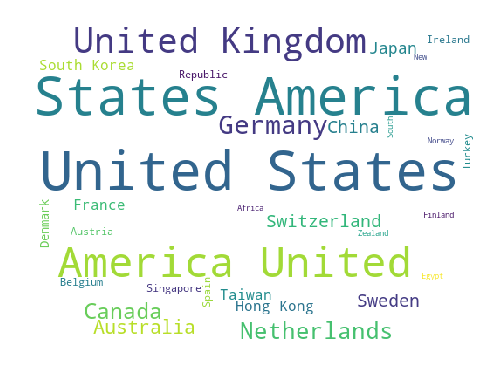# Box Plot¶

In :
# data preparation
x2015 = timesData[timesData.year == 2015]

trace0 = go.Box(
y=x2015.total_score,
name = 'total score of universities in 2015',
marker = dict(
color = 'rgb(12, 12, 140)',
)
)
trace1 = go.Box(
y=x2015.research,
name = 'research of universities in 2015',
marker = dict(
color = 'rgb(12, 128, 128)',
)
)
data = [trace0, trace1]
iplot(data)


# Scatter Plot Matrix¶

In :
# import figure factory
import plotly.figure_factory as ff
# prepare data
dataframe = timesData[timesData.year == 2015]
data2015 = dataframe.loc[:,["research","international", "total_score"]]
data2015["index"] = np.arange(1,len(data2015)+1)
# scatter matrix
fig = ff.create_scatterplotmatrix(data2015, diag='box', index='index',colormap='Portland',
colormap_type='cat',
height=700, width=700)
iplot(fig)


# Inset Plot¶

In :
# first line plot
trace1 = go.Scatter(
x=dataframe.world_rank,
y=dataframe.teaching,
name = "teaching",
marker = dict(color = 'rgba(16, 112, 2, 0.8)'),
)
# second line plot
trace2 = go.Scatter(
x=dataframe.world_rank,
y=dataframe.income,
xaxis='x2',
yaxis='y2',
name = "income",
marker = dict(color = 'rgba(160, 112, 20, 0.8)'),
)
data = [trace1, trace2]
layout = go.Layout(
xaxis2=dict(
domain=[0.6, 0.95],
anchor='y2',
),
yaxis2=dict(
domain=[0.6, 0.95],
anchor='x2',
),
title = 'Income and Teaching vs World Rank of Universities'

)

fig = go.Figure(data=data, layout=layout)
iplot(fig)


# 3D Scatter Plot¶

In :
# create trace 1 that is 3d scatter
trace1 = go